# BMOA-space

Jump to: navigation, search

space of analytic functions of bounded mean oscillation

In 1961, F. John and L. Nirenberg [a4] introduced the space of functions of bounded mean oscillation,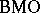, in their study of differential equations (cf. also-space). About a decade later, C. Fefferman proved his famous duality theorem [a1] [a2], which states that the dual of the Hardy space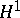is(cf. also Hardy spaces).

In these early works,was studied primarily as a space of real-valued functions, but Fefferman's result raised questions about the nature of analytic functions in the Hardy spaces of the unit disc whose boundary values are in. This is the definition ofand the duality theorem provides the alternative that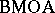consists of those analytic functions that can be represented as a sum of two analytic functions, one with a bounded real part and the other with a bounded imaginary part (cf. also Analytic function; Hardy spaces).

Ch. Pommerenke [a5] proved that a univalent function is inif and only if there is a bound on the radius of the discs contained in the image. Subsequently, W. Hayman and Pommerenke [a3] and D. Stegenga [a7] proved that any analytic function is inprovided the complement of its image is sufficiently thick in a technical sense that uses the notion of logarithmic capacity. As an example, any function whose image does not contain a disc of a fixed radiuscentred at, where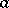,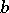range over all integers, satisfies this criterion and hence is in.

In a similar vein, K. Stephenson and Stegenga [a6] proved that an analytic function is inprovided its image Riemann surface (viewed as spread out over the complex plane) has the following property: There are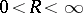,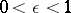so that a Brownian traveller will, with probability at least, fall off the edge of the surface before travelling outward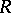units (cf. also Brownian motion). As an example, an overlapping infinite saussage-shaped region can be constructed so that the Riemann mapping function maps onto to the entire complex plane but is nevertheless in.comes up naturally in many problems in analysis, such as on the composition operator, the corona problem (cf. also Hardy classes), and on functions in one and several complex variables.and its variants has become an indispensable tool in real and complex analysis.